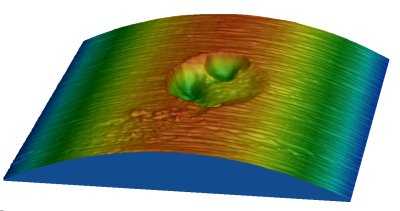In order to determine the different roughness parameters, several operations in the aim of eliminating components which are not pertinent to a given operation need to be carried out.

Surface defects can be classified in three different groups :

• variations of shape, such as cylinders, planes…
• surface waviness,
• surface roughness.The wavelength of the defect determines to which group the defect belongs. In engineering, the maximum wavelength for roughness is thus considered to be 0.8mm.

In order to separate the different components of a surface, several least squares fitting processes have been developed using a geometrical approach. Several filtering processes have been developed using a frequential approach.

### Least Squares Fitting Processes

Global Least Squares Fitting

The principle is to determine and then subtract the average plane from the surface area. To determine the average plane, the least squares fitting method may be used

Selective Least Squares Fitting

This algorithm, which is more sophisticated, is based on automatically eliminating non pertinent zones from a line or from a least squares plane. Curves or surfaces showing significant localized defects can thus be automatically rectified.

Polynomial Least Squares Fitting
The rectification of a plane does not always suffice in eliminating the shape of the sample surface. This is the case for cylindrical, spherical or skew surfaces, for which an average polynomial surface is determined in degrees (n) using the “least squares fitting” method.

### Numerical Filtering

Another means of detecting certain kinds of surface defects ( roughness, waviness, shape) is to use filters.The cut-off frequency is determined in function of the defect under study.

This principle is frequently used by the 2D profile measurement apparatus using analogical filters at a stable frequency (0.8 mm).

The inconvenience of such an analogical filter, other than the choice of the frequency being rather limited, is the fact that its impulse response is asymmetrical, which thus deforms the scan. Numerical filters are thus preferable as they offer an impulse response which is symmetrical in relation to zero.